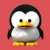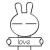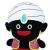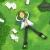## 多对多关系的内存级存储结构

Eviltuzki 发布于 2015/09/18 11:44

1```<?php
header('Content-Type: text/plain; charset=utf-8');
\$student = array(
'3' => array(
'id' => '3',
'name' => '张三'
),
'4' => array(
'id' => '4',
'name' => '李四'
)
);
\$teacher = array(
'5' => array(
'id' => '5',
'name' => '王五'
),
'6' => array(
'id' => '6',
'name' => '赵六'
)
);
\$relation = array(
array(
'student_id' => '3',
'teacher_id' => '5',
'course' => 'PHP Web开发'
),
array(
'student_id' => '4',
'teacher_id' => '5',
'course' => 'PHP Web开发'
),
array(
'student_id' => '3',
'teacher_id' => '4',
'course' => 'MySQL应用和开发'
)
);
//查询id为3的学生的课程和任课老师
\$id = 3;
\$arr = array(
'id' => \$id,
'name' => \$student[\$id]['name']
);
foreach(\$relation as \$k => \$v) {
if(\$v['student_id']==\$id) {
\$arr['course'][\$k]['teacher_id'] = \$v['teacher_id'];
\$arr['course'][\$k]['course'] = \$v['course'];
}
}
print_r(\$arr);
//输出:
Array
(
[id] => 3
[name] => 张三
[course] => Array
(
 => Array
(
[teacher_id] => 5
[course] => PHP Web开发
)

 => Array
(
[teacher_id] => 4
[course] => MySQL应用和开发
)

)

)```= =你这个PHP的例子感觉就是多对多数据库关系额。。。。第一种方案直接否了。。笔试这么答，肯定死。。。 哈希我只知道相当于一个变相的索引，你这个PHP例子就是哈希么？
00